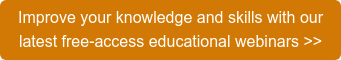# Liquid Chromatography Blog

In the next few blogs in this HPLC Solutions serieswe’ll look at several aspects of the use of internal standards (IS) in HPLC and LC-MS applications. The topic of this discussion is how an internal standard is used in quantitative analysis.

An internal standard is used to aid quantification of an analyte, especially when volumetric loss of the sample is probable, as with a multi-step sample preparation scheme. In concept it is quite simple: you add a constant amount of the internal standard to every sample, and instead of keeping track of absolute peak area or height for calibration purposes, you use the ratio of the area or height of the analyte and internal standard. For example, consider the blue chromatogram inset in the figure. The normal sample is shown as the peaks comprising lines. To this sample, a known amount of another compound (represented by the solid peak) is added as an internal standard. The same process is used to prepare the calibration samples: calibrators at different concentrations each have the same amount of internal standard added to them. When the chromatogram is integrated, the peak areas of the analyte and the internal standard are measured. The ratio of these areas is then used in the calibration process.The calibration plot is made first, as shown in the figure. We know the concentration of each calibrator (Cc) and the concentration of internal standard added (Cis) as well as the area of the peak for that calibrator (Ac) and the area for the internal standard in that calibrator (Ais). Let’s say we make calibrators at 10, 20, 50, and 100 ng/mL with 20 ng/mL of IS in each. After the calibration samples are run, we’ll have a series of paired areas corresponding to the paired concentrations. Take the ratio of each, Cc,10/Cis, Ac,10/Ais, Cc,20/Cis, Ac,20/Ais, etc., where the numbered subscripts correspond to the concentrations and areas for the 10 ng/mL, 20 ng/mL calibrator, and so forth. Now these can be plotted as shown by the green arrows in step #1 of the figure, where the concentration ratio is plotted on the x-axis and the area ratio for that sample is plotted on the y-axis. This will then form the calibration line for the sample batch. When unknown samples are run, the area of the analyte and internal standard is measured and the ratio is determined, which allows you to determine what concentration ratio this represents, as shown by the purple arrows in step #2 of the figure.

This process sounds rather complex, so you might ask why we would use internal standard calibration. Assume that you are doing a liquid-liquid extraction of your sample, followed by evaporation to dryness of an organic phase, and reconstitution of the residue in the injection solvent. The internal standard helps to correct for uncontrollable changes in the sample volume along the way. If the IS is added prior to the extraction step, you don’t have to worry so much about the volumetric recovery from the extraction step. For example, if you recover the top phase by drawing it off with a pipette, it doesn’t matter if you remove 70% or 80% of the volume of that phase, because the ratio of the analyte to internal standard will remain constant, independent of the absolute volume you recover. Similarly, if you make a small error in the amount of injection solvent added to redissolve the residue, it won’t matter, because the ratio of analyte to internal standard will remain the same, regardless of whether you add 95 µL or 105 µL of solvent.

We have seen that internal standards can be used to help correct for volumetric recovery errors in sample preparation. By adding a constant amount of IS to every calibrator, we can construct a calibration curve (red line in the figure) based on the concentration and area ratios of calibrator to internal standard (green line). Similarly, adding the same amount of IS to every sample, and tracking the ratio of analyte to internal standard peak area, we can then use the calibration curve to determine the amount of analyte present in each sample (purple line). Of course, the data system is directed to make the calibration plots and determine analyte concentrations in our unknown samples so we don’t have to do the work manually.This blog article series is produced in collaboration with John Dolan, best known as one of the world’s foremost HPLC troubleshooting authorities. He is also known for his ongoing research with Lloyd Snyder, resulting in more than 100 technical publications and three books. If you have any questions about this article send them to TechTips@sepscience.com